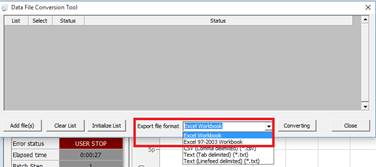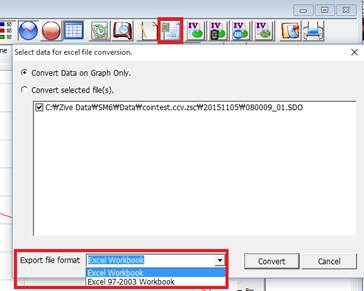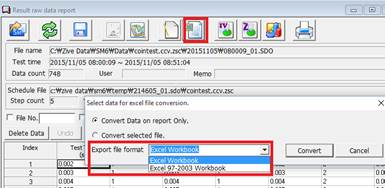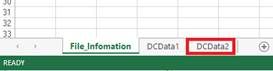# Excel Converting

This function will convert data to Excel format.

You can convert your data file to Excel format in the following menu.

## 1.       Tool-data conversionThis menu  can convert multiple data files to excel files

## 2.       Graph-Excel convertingYou can convert graph data to excel file on graph display.

## 3.       Data Editor-Excel convertingYou can convert report data to excel file on report display.

## 4.       Excel converting Function

This provides two kind of excel format.

·         Excel binary workbook format:(xlsb) Excel97 or higher version

·         Excel workbook format(xls) Excel97-2003

If you use WindowsXP SP3, then you must select Excel97-2003 when you convert your data to excel.

If you select “convert current view”, each specified axis value will be converted to excel format. If you select “convert selected file”, the selected file(s) will be converted to excel file(s) with all data included in the data file.

Excel converting process uses the external program, ConvertingExcel6.exe, which is in “c:\program files\zivelab\sm (64bit OS)” or “c:\program files(x86)\zivelab\sm (32bit OS) ” folder.

It works as background operation.

For converting Excel97-2003 format(xls), maximum row per sheet is 65535. If the data points is larger than 65534, new sheet will be created for over 65534 points display.

For converting Excel binary workbook format (xlsb), the maximum row per sheet is 1,048,576 rows, If the data points is larger than 1,048,575, new sheet will be created for over 1,048,575 points display